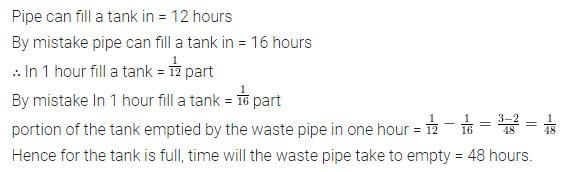# ML Aggarwal Class 8 Solutions for ICSE Maths Chapter 9 Direct and Inverse Variation Ex 9.3

## ML Aggarwal Class 8 Solutions for ICSE Maths Chapter 9 Direct and Inverse Variation Ex 9.3

Question 1.
A farmer can reap a field in 10 days while his wife can do it in 8 days (she does not waste time in smoking). If they work together, in how much time can they reap the field?
Solution: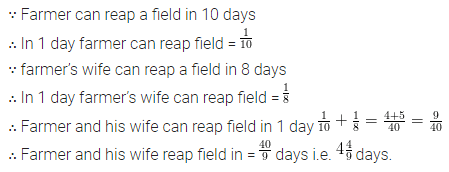Question 2.
A can-do $$\frac{1}{5}$$th of a certain work in 2 days and B can do $$\frac{2}{3}$$rd of it in 8 days. In how much time can they together complete the work?
Solution:Question 3.
One tap fills a tank in 20 minutes and another tap fills it in 12 minutes. The tank being empty and if both taps are opened together, in how many minutes the tank will be full?
Solution: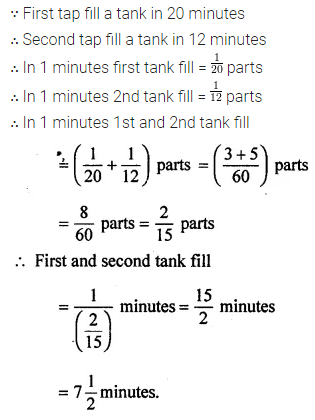Question 4.
A can do a work in 6 days and B can do it in 8 days. They worked together for 2 days and then B left the work. How many days will A require to finish the work?
Solution: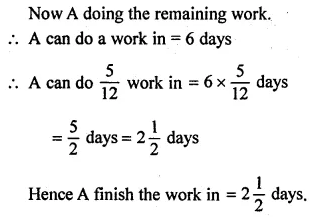Question 5.
A can do a piece of work in 40 days. He works at it for 8 days and then B finishes the remaining work in 16 days. How long will they take to complete the work if they do it together?
Solution:Question 6.
A and B separately do a work in 10 and 15 Solution: days respectively. They worked together for some days and then A completed the remaining work in 5 days. For how many days had A and B worked together?
Solution: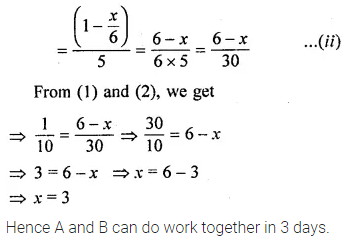Question 7.
If 3 women or 5 girls take 17 days to complete a piece of work, how long will 7 women and 11 girls working together take to complete the work?
Solution:Question 8.
A can do a job in 10 days while B can do it in 15 days. If they work together and earn ₹3500, how should they share the money?
Solution: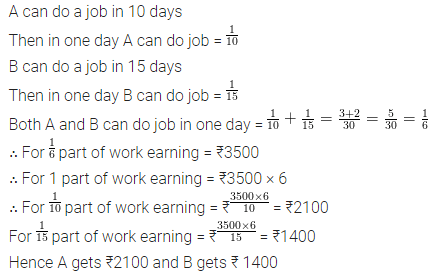Question 9.
A, B and C can separately do a work in 2, 6 and 3 days respectively. Working together, how much time would they require to do it? If the work earns them ₹1960, how should they divide the money?
Solution: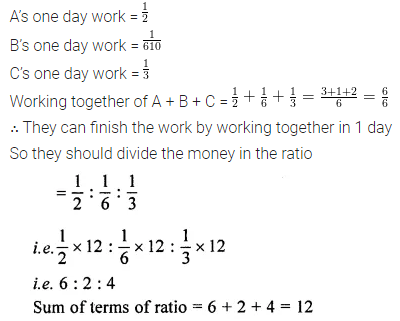Question 10.
A, B and C together can do a piece of work in 15 days, B alone can do it in 30 days and C alone can do it in 40 days. In how many days will A alone do the work?
Solution:Question 11.
A, B and C working together can plough a field in $$4 \frac{4}{5}$$ days. A and C together can do it in 8 days. How long would B working alone take to plough the field?
Solution: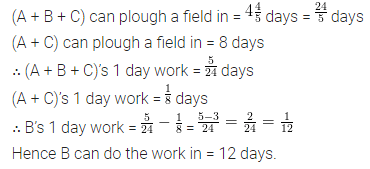Question 12.
A and B together can build a wall in 10 days; B and C working together can do it in 15 days; C and A together can do it in 12 days. How long will they take to finish the work, working altogether? Also find the number of days taken by each to do the ^ame work, working alone.
Solution: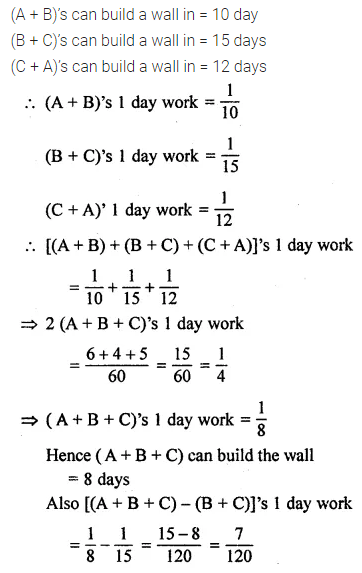Question 13.
A pipe can fill a tank in 12 hours. By mistake, a waste pipe in the bottom is left opened and the tank is filled in 16 hours. If the tank is full, how much time will the waste pipe take to empty it?
Solution:.